Custom SearchPRACTICE PROBLEMS: Find the equations of the tangent line and the normal line and the lengths of the tangent and the normal for each of the following curves at the point indicated: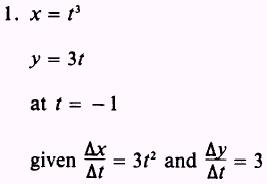4. Find the points of contact of the horizontal and the vertical tangents to the curvegiven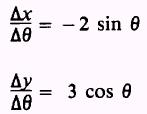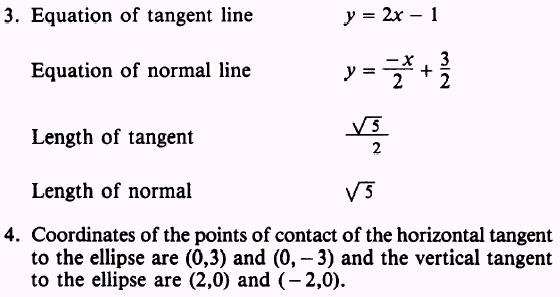SUMMARY The following are the major topics covered in this chapter: 1. Slope of a curve at a point:whereequals the inclination of the tangent line. If the line tangent to the curve is horizontal, thenIf the line tangent to the curve is vertical, thenWhen the slope of a curve is zero, the curve may be at either a maximum or a minimum. 2. Tangent at a given point on the standard parabola y2 = 4ax: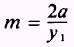where a is the same as in the standard equation for parabolas, and y1 is the y coordinate of the given point (x1,y1). 3. Tangent at a given point on other curves: To find the slope, m, of a given curve at point P1(x1,y1), choose a second point, P', on the curve so that it has coordinates (x1+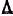X,y1 +y); then substitute each of the coordinates of P' and P1 in the equation of the curve and simplify. Divide both sides byx and eliminate terms that contain powers ofy higher than the first power. Solve forLetx approach zero and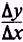will approach the slope of the tangent line, m, at point P1. 4. Equation of the tangent line: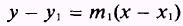5. Equation of the normal line: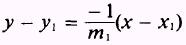6. Relationships between the slopes of the tangent and normal lines: The slope of the normal line is the negative reciprocal of the slope of the tangent line. The inclination of one line must be 90 greater than the other. 7. Length of the tangent: The length of the tangent is defined as that portion of the tangent line between the point P,(x,,y,) and the point where the tangent line crosses the X axis. length of the tangent =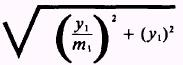8. Length of the normal: The length of the normal is defined as that portion of the normal line between the point P1 (x1,y1) and the X axis. length of the normal =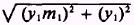9. Parametric equations: If the variables x and y of the Cartesian coordinate system are expressed in terms of a third variable, say t (or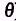), then the variable t (or) is called a parameter. The two equations x = x(t) and y = y(t) [or x = x() and y = y()] are called parametric equations.Integrated Publishing, Inc. - A (SDVOSB) Service Disabled Veteran Owned Small Business